# Even/odd numbers

What is the product of 0.97 and the next odd decimal number?

x =  0.97

### Step-by-step explanation: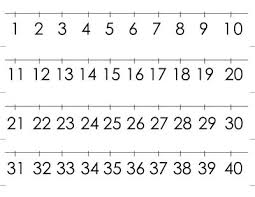Did you find an error or inaccuracy? Feel free to write us. Thank you!## Related math problems and questions:

• Two numbers and its productThe product of two numbers are 2/3. If on of them is 1/10, what is the other?
• DigitsHow many odd four-digit numbers can we create from digits: 0, 3, 5, 6, 7? (a) the figures may be repeated (b) the digits may not be repeated
• Without 2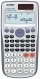Without multipying, tell whether the product 0.644 x 0.25 will be greater than 1 or less than 1? Explane how you know. Then find the product.
• Cash back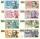After a trip 24 students have left more than 650 but less than 690 CZK (Kc). How much each student receives return when the amount is divisible by the number of pupils?
• David numberJana and David train the addition of the decimal numbers so that each of them will write a single number and these two numbers then add up. The last example was 11.11. David's number had the same number of digits before the decimal point, the Jane's numbe
• Prime factorsWrite 98 as a product of prime factors
• Prime factorsFactor the number 6600 into the product of prime numbers.
• DivisibilityDetermine all divisors of number 84.
• Even / odd numbers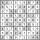a / Using variable n write two consecutive odd numbers. b / the sum of three consecutive odd numbers is 333. What are this numbers?
• Street numbersLada came to aunt. On the way he noticed that the houses on the left side of the street have odd numbers on the right side and even numbers. The street where he lives aunt, there are 5 houses with an even number, which contains at least one digit number 6
• Find unknown numberWhat is the number between 50 and 55 that is divisible by 2,3,6,9?
• The largest numberFind the largest integer such that: 1. No figures is not repeat, 2. multiplication of every two digits is odd, 3. addition all digits is odd.
• Odd numbersThe sum of four consecutive odd numbers is 1048. Find those numbers ...
• Closest natural numberFind the closest natural number to 4.456 to 44.56 and 445.6.
• Perpetrator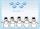The perpetrator is a number that is smaller than the number 80. It is a multiple of five and it is odd. If we added up the tens and ones of this number we get number 8.
• The sumThe sum of five consecutive odd numbers is 75. Find out the sum of the second and fourth of them.
• NumberWhat number should be placed instead of the asterisk in number 702*8 to get a number divisible by 6?